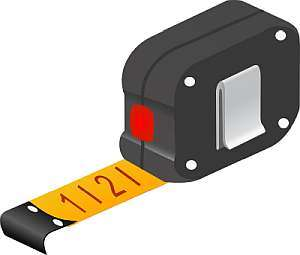# Feet and Inches to inches calculator

Instructions: Use this calculator to convert a lengths or heights given in feet+inches notation into inches, showing all the steps. Please type a length you would like to convert in the form below such 6'2", etc:Type a length in feet and inches (Ex: 6' 1")

## Using this Feet+Inches to Inches Conversion Calculator

This calculator allows you to take lengths and heights given in feet+inches, and to convert them into inches. The process is simple, and it is really not a conversion per se, as we are translating units from within the same unit system (the imperial notation).

Still, this kind of conversion of heights to inches is quite common, and therefore, you can use this calculator for that purpose.## How can you convert feet+inch lengths into inches?

In order to conduct this kind of conversion, the basic starting point is by understanding that a measure like 5' 11" is intended to have two parts: 5 feet and 11 inches. So you can convert the feet to inches, and then add it to the number of inches provided (if any).

## How do you do the height conversion when you have imperial notation

As you indicated above, we need to separate the amount given in imperial notation into feet and inches, and then use a conversion formula for feet.

### Steps for the conversion of feet+inches to inches

1. We observe that that 1 foot is 12 inches. This is used as the initial proportion
2. Now, for a length or height that is given in feet+inches format, you identify the number of feet F, and the number of inches I* that are given
3. We use direct proportion to establish that $$\displaystyle \frac{1}{12} = \frac{F}{I*}$$
4. Solving the proportion we get the following conversion formula for inches: $$I = 12 F + I*$$

Then by using this formula we can conduct a general conversion by identifying the number of feet $$F$$ and inches $$I*$$ that are given for the conversion.### Examples: Converting imperial units to inches

What is 5'2 in inches?

Solution: Let us take a look at the formula for the conversion:

$I = 12 F + I*$

In this case, the given length consists of 5 feet and 2 inches, so then plugging it in the above formula we find that :

$I = 12 F + I* = 12 \times 5 + 2 = 62 \text{ inches}$

### Another conversion example

Convert 5'7 in inches

Solution: Same as how we did it above, we need to take a look at the conversion formula. We have in this case F = 5 and I* = 7:

$I = 12 F + I*$

and now the given length is 5' 9, which corresponds to 5 feet and 9 inches, so we find that:

$I = 12 F + I* = 12 \times 5 + 7 = 67 \text{ inches}$

### More unit conversions

Convert 5'11" in inches

Solution: We need to take again a look at the formula:

$I = 12 F + I*$

In this case we have 5' 1", which is decomposed as 6 feet and 2 inches. Therefore, so we find that:

$I = 12 F + I* = 12 \times 5 + 11 = 71 \text{ inches}$

## Other interesting metric calculators

Our site provides a number of metric conversion that can be useful to you, for example, our meters to inches calculator, or the centimeters to feet calculator, or also our meters to feet, or imperial to meters calculators. You can choose among those based on what specific conversion you need to conduct.